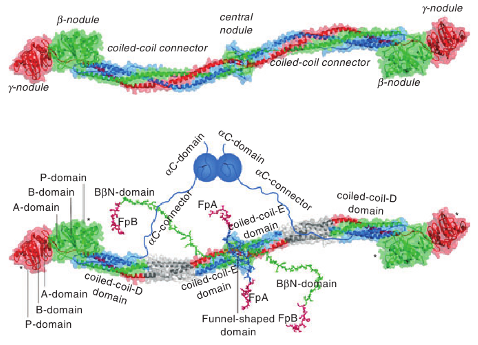# Mechanical properties of protein fibers¶

We have been working with the experimental group of Dr. Weisel (University of Pennsylvania, School of Medicine) to resolve the structural details and the mechanism of force-induced unfolding of fibrinogen monomer $$Fb$$ and oligomers $$(Fb)_n$$ (see Figure). Although the physical properties of fibrin fibers, the major structural component of a blood clot, which control their function in hemostasis and wound healing, are have been fully characterized, the underlying mechanism of their force-driven elongation is not understood. We carry out computational studies of the mechanical properties of fibrin protofibrils on GPUs using the SOP-GPU package, in order to characterize the micromechanics of fibrin at the monomer, oligomer, and fiber level. We use GPU-based computations to speedup the molecular simulations. For example, it takes ~15 days to obtain one trajectory of the mechanical unfolding for the oligomer $$(Fb)_3$$ of three $$Fb$$ repeats on a GPU GeForce GTX 480, using the SOP model implemented on a GPU (SOP-GPU). For comparison, it would take ~12 years of the wall-clock time to complete a single simulation run on a single CPU core of comparable level of technology, using the same SOP model.

The results were published in Structure (2010).Figure: Fibrinogen structure. Left panel: Crystal structure of fibrinogen. The central nodule, formed by the $$N$$-terminal portions of all six chains, is connected to the distal $$\beta$$- and $$\gamma$$-nodules formed by the $$C$$-terminal portions of the $$B\beta$$ and $$\gamma$$ chains, respectively, by triple-helical coiled-coils, each formed by the middle portions of the $$A\alpha$$, $$B\beta$$ and $$\gamma$$ chains. Right panel: Same molecule as on top but with those regions that are not identified in the crystal structure, the interacting $$\alpha C$$-domains attached to the molecule with the ﬂexible $$\alpha C$$-connectors, and the $$N$$-terminal portions of the $$B\beta$$ chains forming the functional $$B\beta N$$-domains. The funnel-shaped domain in the center contains fibrinopeptides A (FpA) and fibrinopeptides B (FpB); the $$\gamma N$$-domain is located on the opposite side of the molecule (not shown). The individual domains of the D regions, i.e., A-domain, B-domain, and P-domain, are indicated only in one subunit of the molecule. The site “a” (hole “a”) and site “b” (hole “b”) in the P-domain of the $$\gamma$$- and $$\beta$$-nodules, respectively, are indicated by asterisks. (taken from J.Thromb.Haemost 7: 355 (2009)).

## Forced unfolding in silico of the fibrinogen monomers and oligomers¶

Large-size protein systems unfold through the gradual detachment of two or several subdomain and/or through the simultaneous or sequential unraveling of the various secondary structure elements. This allows us to utilize coarse-grained descriptions of biomolecules to describe the global mechano-chemical unfolding reactions. We utilize the SOP-GPU package to carry out Langevin simulations of fibrinogen monomer $$Fb$$ (~2,000 residues) and dimer $$(Fb)_2$$ (~4,000 residues) using the experimental force-ramp conditions, $$f(t)=r_ft$$, where $$r_f=\kappa \nu_f$$ is the force-loading rate. We use the experimentally relevant values of the pulling speed $$\nu_f=1$$ $$\mu m/s$$ and the cantilever spring constant $$\kappa=35$$ pN/nm. To obtain a single trajectory of unfolding for $$Fb$$, the system dynamics should be propagated numerically over as many as $$5 \times 10^9$$ iterations (0.2 seconds of real time). To fully utilize the GPU computational resources, we employ the multiple-runs-per-GPU approach, which allows to run many trajectories for the system under the study concurrently on a single GPU. It takes ~17 day to generate 5 trajectories on a GPU GeForce GTX 295 (NVIDIA). For comparison, it would take ~18 months of wall-clock time to complete a single simulation run on a CPU of a similar level of technology. It turns out that the unfolding micromechanics and the corresponding dynamic signatures in the force spectra change dramatically with increased pulling speed ($$\nu_f=2.5$$ and $$25$$ $$\mu m/s$$).